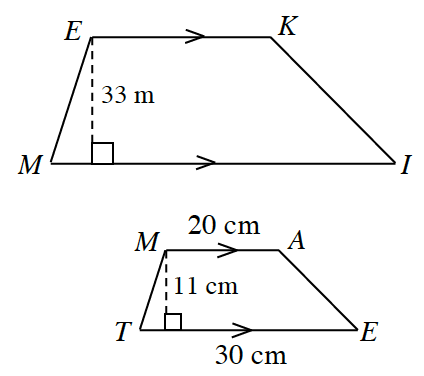### Home > INT2 > Chapter 9 > Lesson 9.3.3 > Problem9-112

9-112.The trapezoids at right are similar.

1. What is the ratio of the heights? Hint: It is not $3$ or $\frac { 1 } { 30 }$.

Refer to the Math Notes box.

2. Compare the areas. What is the ratio of the areas?

Use proportional equations to find the missing dimensions.
Refer to the Math Notes box.

The formula to find the area of a trapezoid is $\frac{1}{2}h(b_1+b_2)$